Tamilnadu State Board New Syllabus Samacheer Kalvi 12th Business Maths Guide Pdf Chapter 7 Probability Distributions Ex 7.4 Text Book Back Questions and Answers, Notes.

## Tamilnadu Samacheer Kalvi 12th Business Maths Solutions Chapter 7 Probability Distributions Ex 7.4

Question 1.
Normal distribution was invented by
(a) Laplace
(b) De-Moivre
(c) Gauss
(d) all the above
Solution:
(b) DemoivreQuestion 2.
If X ~ N(9, 81) the standard normal variate Z will be
(a) Z = $$\frac { X-81 }{9}$$
(b) Z = $$\frac { X-9 }{81}$$
(c) Z = $$\frac { X-9 }{9}$$
(d) Z = $$\frac { 9-X }{9}$$
Solution:
(b) Z = $$\frac { X-9 }{81}$$
Hint:
Here µ = 9
σ² = 81
∴ σ = 9
Z = $$\frac { X-µ }{σ}$$ = $$\frac { X-9 }{9}$$

Question 3.
If Z is a standard normal variate, the proportion of items lying between Z = -0.5 and Z = -3.0 is
(a) 0.4987
(b) 0.1915
(c) 0.3072
(d) 0.3098
Solution:
(c) 0.3072
Hint: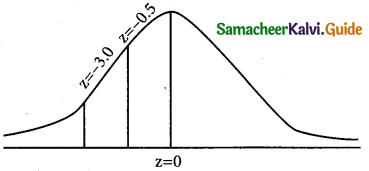p(-3.0 < z < -0.5)
= p(0.5 < z < 3.0) – p(0 < z < o.5)
= 0.4987 – 0.1915 = 0.3072

Question 4.
If X ~ N(µ, σ2), the maximum probability at the point of inflexion of normal distribution
(a) ($$\frac { 1 }{\sqrt{2π}}$$)e$$\frac { -1 }{2}$$
(b) ($$\frac { 1 }{\sqrt{2π}}$$)e$$\frac { 1 }{2}$$
(c) ($$\frac { 1 }{σ\sqrt{2π}}$$)e$$\frac { -1 }{2}$$
(d) ($$\frac { 1 }{\sqrt{2π}}$$)
Solution:
(c) ($$\frac { 1 }{σ\sqrt{2π}}$$)e$$\frac { -1 }{2}$$

Question 5.
In a parametric distribution, the mean is equal to variance is
(a) binomial
(b) normal
(c) Poisson
(d) all the above
Solution:
(c) Poisson

Question 6.
In turning out certain toys in a manufacturing company, the average number of defectives is 1%. The probability that the sample of 100 toys there will be 3 defectives is
(a) 0.0613
(b) 0.613
(c) 0.00613
(d) 0.3913
Solution:
(a) 0.0613
Hint:
Given
p = 0.01 and n = 100
λ = np = 0.01 × 100 = 1
p(x = x) = $$\frac { e^{-λ}λ^x }{x!}$$
p(x = x) = $$\frac { e^{-1}(1)^3 }{3!}$$ = $$\frac { 0.3678 }{6}$$ = 0.0613Question 7.
The parameters of the normal distribution
f(x) ($$\frac { 1 }{\sqrt{72π}}$$) $$\frac { e^{-(x-10)^2} }{72}$$ – ∞ < x < ∞
(a) (10, 6)
(b) (10, 36)
(c) (6, 10)
(d) (36, 10)
Solution:
(b) (10, 36)
Hint:Here σ = 6 and µ = 10
∴ σ = (6)² = 36

Question 8.
A manufacturer produces switches and experiences that 2 percent of switches are defective. The probability that in a box of 50 switches, there are at most two defective is:
(a) 2.5 e-1
(b) e-1
(c) 2 e-1
(d) none of the above
Solution:
(a) 2.5 e-1
Hint:Question 9.
An experiment succeeds twice as often as it fails. Die chance that in the next six trials, there shall be at least four successes is
(a) 240/729
(b) 489/729
(c) 496/729
(d) 251/729
Solution:
(c) 496/729
Hint:
p = 2 q ⇒ p = 2(1 – p)
p (2 – 2p) ⇒ 3p = 2
p = 2/3 and q = 1 – p ⇒ q = 1 – 2/3
q = 1/3 and n = 6
p(X = x) = ncxpxqn-x
p(x ≥ 4) = p(x = 4) + p(x = 5) + p(x = 6)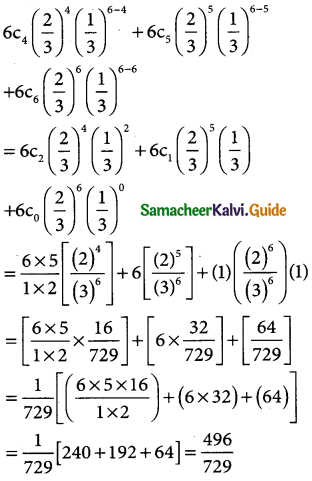Question 10.
If for a binomial distribution b(n, p) mean = 4 and variance = 4/3, the probability, P(X ≥ 5) is equal to:
(a) (2/3)6
(b) (2/3)5( 1/3)
(c) (1/3)6
(d) 4(2/3)6
Solution:
(d) 4(2/3)6
Hint:
In a binomial distribution
mean np = 4 → (1)
variance npq = 4/3 → (2)
(2) ÷ (1) ⇒ npq = 4/3 ⇒ q = 1/3
p = (1 – q) ⇒ p = 1 – 1/3
∴ p = 2/3
p(X = x) = ncrpxqn-x
p(x ≥ 5) = p(x = 5) + p(x = 6)Question 11.
The average percentage of failure in a certain examination is 40. The probability that out of a group of 6 candidates atleast 4 passed in the examination are:
(a) 0.5443
(b) 0.4543
(c) 0.5543
(d) 0.4573
Solution:
(b) 0.4543
Hint:
given:
n = 6
q = 40/100 = 2/5
p = 1 – q = 1 – 2/5 = 3/5
p(X = x) = ncxpxqn-x
p(x ≥ 4) = p(x = 4) + p(x = 5) + p(x = 6)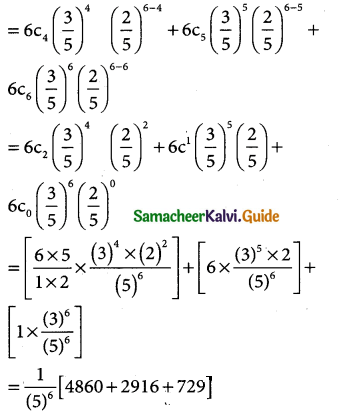= $$\frac { 8505 }{5^6}$$ = $$\frac { 1701 }{3125}$$
= 0.54432

Question 12.
Forty percent of the passengers who fly on a certain route do not check in any luggage. The planes on this route seat 15 passengers. For a full flight, what is the mean number of passengers who do not check in any luggage?
(a) 6.00
(b) 6.45
(c) 7.20
(d) 7.50
(a) 6.00
Hint:
n = 15, p = 0.4
⇒ mean (np) = 6

Question 13.
Which of the following statements is/are true regarding the normal distribution curve?
(a) it is a symmetrical and bell-shaped curve
(b) it is asymptotic in that each end approaches the horizontal axis but never reaches it
(c) its mean, median, and mode are located at the same point
(d) all of the above statements are true.
Solution:
(d) all of these above statements are true

Question 14.
Which of the following cannot generate a Poisson distribution?
(a) The number of telephone calls received in a ten-minute interval
(b) The number of customers arriving at a petrol station
(c) The number of bacteria found in a cubic foot of soil
(d) The number of misprints per page
Solution:
(b) The number of customers arriving a petrol stationQuestion 15.
The random variable X is normally distributed with a mean of 70 and a standard deviation of 10. What is the probability that X is between 72 and 84?
(a) 0.683
(b) 0.954
(c) 0.271
(d) 0.340
Solution:
(d) 0.340
Hint:
In a normal distribution
S.D(σ) = 10
mean(µ) = 70 and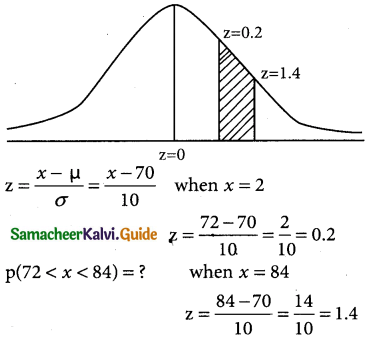= p(0.2 < z < 1.4) – p(0 < z < 0.2)
= 0.4192 – 0.0793
= 0.3399

Question 16.
The starting annual salaries of newly qualified chartered accountants (CA’s) in South Africa follow a normal distribution with a mean of Rs 180,000 and a standard deviation of Rs 10,000. What is the probability that a randomly selected newly qualified CA will earn between Rs 165,000 and Rs 175,000 per annum?
(a) 0.819
(b) 0.242
(c) 0.286
(d) 0.533
Solution:
(b) 0.242
Hint:
In a normal distribution
µ = 180,000 and σ = 10,000
z = $$\frac { X-µ }{σ}$$ = $$\frac { X-180000 }{10000}$$
p(165,000 < x < 1,75,000) = ?
when x = 165,000
z = $$\frac { 165000-180000 }{10000}$$ = $$\frac { 15000 }{10000}$$
z = -1.5when x = 175,000
z = $$\frac { 175000-180000 }{10000}$$ = $$\frac { -5000 }{10000}$$ = $$\frac { -1 }{2}$$
z = -0.5
p(165,000 < x < 175,000)
= p(-1.5 < z < -0.5)
= p(0.5 < z < 1.5)
= p(0 < z < 1.5) – p(0 < z < 0.5)
= 0.4332 – 0.1915 = 0.2417

Question 17.
In a large statistics class the heights of the students are normally distributed with a mean of 172 cm and a variance of 25 cm. What proportion of students are between 165 cm and 181 cm in height?
(a) 0.954
(b) 0.601
(c) 0.718
(d) 0.883
Solution:
(d) 0.883
Hint:In a normal distribution
µ = 172; σ² = 25 then σ = 5
z = $$\frac { x-µ }{σ}$$ = $$\frac { x-172 }{5}$$
p(165 < x < 181) = ?
when x = 165 z = $$\frac { 165-172 }{5}$$ = $$\frac { -7 }{5}$$ = -1.4
when x = 181 z = $$\frac { 181-172 }{5}$$ = $$\frac { 9}{5}$$ = 1.8
p(165 < x < 181) = p(-1.4 < z < 1.8)
= p(-1.4 < z < 0) + p(0 < z < 1.8)
= p(0 < z < 1.4) + p(0 < z < 1.8)
= 0.4192 + 0.4641
= 0.8833Question 18.
A statistical analysis of long-distance” telephone calls indicates that the length of these calls is normally distributed with a mean of 240 seconds and a standard deviation of 40 seconds. What proportion of calls lasts less than 180 seconds?
(a) 0.214
(b) 0.094
(c) 0933
(d) 0.067
Solution:
(d) 0.067
Hint:
In a normal distribution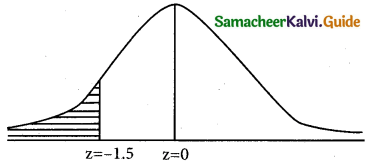µ = 240 and σ = 40Question 19.
Cape town is estimated to have 21% of homes whose owners subscribe to the satellite service, DSTV. If a random sample of your home is taken, what is the probability that all four homes subscribe to DSTV?
(a) 0.2100
(b) 0.5000
(c) 0.8791
(d) 0.0019
Solution:
(d) 0.0019
Hint:
p = $$\frac { 21 }{100}$$ = 0.21
p(x = 4) = (0.21) × (0.21) × (0.21) × (0.21)
= 0.00194481

Question 20.
Using the standard normal table, the sum of the probabilities to the right of z = 2.18 and to the left of z = -1.75 is:
(a) 0.4854
(b) 0.4599
(c) 0.0146
(d) 0.0547
Solution:
(d) 0.0547
Hint:p(z < – 1.75) + p(z > 2.18)
= p(-∞ < z < 0) – p(-1.75 < z < 0) + p(0 < z < ∞) – p(0 < z < 2.19)
= 0.5 – p(0 < z < 1.75) + 0.5 – p(0 < z < 2.18)
= (0.5 – 0.4599) + (0.5 – 0.4854)
= 0.0401 + 0.0146
= 0.0547

Question 21.
The time until the first failure of a brand of inkjet printers is normally distributed with a mean of 1,500 hours and a standard deviation of 200 hours. What proportion of printers fails before 1000 hours?
(a) 0.0062
(b) 0.0668
(c) 0.8413
(d) 0.0228
Solution:
(a) 0.0062
Hint: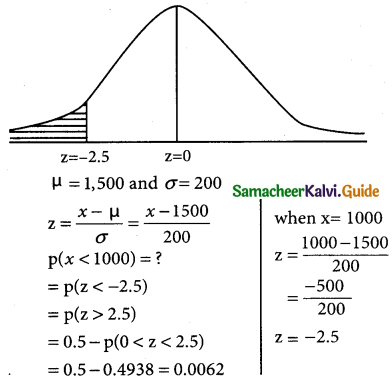Question 22.
The weights of newborn human babies are normally distributed with a mean of 3.2 kg and a standard deviation of 1.1 kg. What is the probability that a randomly selected newborn baby weighs less than 2.0 kg?
(a) 0.138
(b) 0.428
(c) 0.766
(d) 0.262
Solution:
(a) 0.138
Hint:Question 23.
Monthly expenditure on their credit cards, by credit cardholders from a certain bank, follows a normal distribution with a mean of Rs 1,295.00 and a standard deviation of Rs 750.00. What proportion of credit cardholders spend more than Rs 1,500.00 on their credit cards per month?
(a) 0.487
(b) 0.392
(c) 0.500
(d) 0.791
Solution:
(b) 0.392
Hint:
In a normal distributionmean m = 1295 and S.D σ = 7.50
p(x > 1500) = ?
when x = 1500
z = $$\frac { 1500-1295 }{750}$$ = $$\frac { 205 }{750}$$ = 0.273
(ie) p(x > 1500) = p(z > 0.273)
= 0.5 – p(0 < z < 0.273)
= 0.5 – 0.1064
= 0.3936

Question 24.
Let z be a standard normal variable. If the area to the right of z is 0.8413, then the value of z must be:
(a) 1.00
(b) -1.00
(c) 0.00
(d) -0.41
Solution:
(b) -1.00
Hint: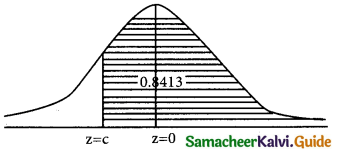p(-c < z < ∞) = 0.8413
p(-c < z < 0) + 0.5 = 0.8413
p(-c < z < 0) = 0.813 – 0.5 = 0.3413
p(0 < z < c) = 0.3413 ⇒ c = 1.00
∴ -c = -1.00

Question 25.
If the area to the left of a value of z (z has a standard normal distribution) is 0.0793, what is the value of z?
(a) -1.41
(b) 1.41
(c) -2.25
(d) 2.25
Solution:
(a) -1.41
Hint: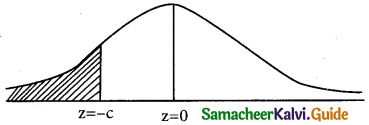p(z < -c) = 0.0793 ie p(z > c) = 0.0793
p(0 < z < ∞) – p(0 < z < c) = 0.0793
0. 5 – p(0 < z < c) = 0.0793
p(0 < z < c) = 0.5 – 0.0793
p(0 < z < c) = 0.4207
c = 1.41
then -c = -1.41Question 26.
If P(Z > z) = 0.8508 what is the value of z (z has a standard normal distribution)?
(a) -0.48
(b) 0.48
(c) -1.04
(d) 1.04
Solution:
(c) -1.04
Hint:
since the area is greater than 0.5 then the z must be negative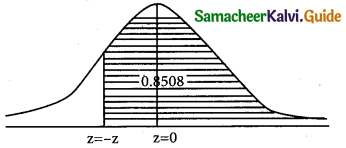p(z < Z) = 0.8508
p(-z < Z < 0) + p(0 < z < ∞) = 0.8508
p(-z < Z < 0) + 0.5 = 0.8508
p(-z < Z < 0) = 0.8508 – 0.5 = 0.3508
p(0 < Z < z) = 0.3508
z = 1.04 then -z = -1.04

Question 27.
If P(Z > z) = 0.5832 what is the value of z (z has a standard normal distribution)?
(a) -0.48
(b) 0.48
(c) 1.04
(d) -0.21
Solution:
(d) -0.21
Hint:
p(z > Z) = 0.5832
since area >0.5 then then z must be negative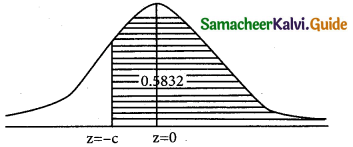p(- z < Z < ∞) = 0.5832
p(-z < Z < 0) + p(0 < Z < ∞) = 0.5832
p(-z < Z < 0) + 0.5 = 0.5832
p(-z < Z < 0) = 0.0832
z = 0.21
then -z = -0.21

Question 28.
In a binomial distribution, the probability of success is twice that of failure. Then out of 4 trials, the probability of no success is
(a) 16/81
(b) 1/16
(c) 2/27
(d) 1/81
Solution:
(d) 1/81
Hint:
In a binomial distribution
p = 2q ⇒ p = 2(1 – p)
p = 2 – 2p ⇒ 3p = 2 ⇒ p = 2/ 3
q = 1 – p = 1 – 2/3
∴ q = 1/3
p(X = x) = ncxpxqn-x = 4cx ($$\frac { 2 }{3}$$)x ($$\frac { 1 }{3}$$)4-x
p(X = 0) = 4c0 ($$\frac { 2 }{3}$$)0 ($$\frac { 1 }{3}$$)4-0
= (1)(1)($$\frac { 1 }{3}$$)4 = $$\frac { 1 }{81}$$